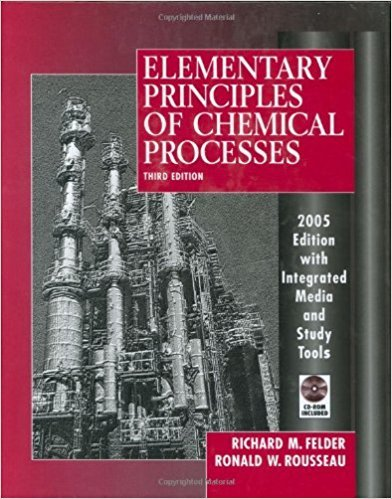×
Get Full Access to Elementary Principles Of Chemical Processes - 3 Edition - Chapter 14 - Problem 14.12
Get Full Access to Elementary Principles Of Chemical Processes - 3 Edition - Chapter 14 - Problem 14.12

×

# For the required coal feed rate, scale the results fromISBN: 9780471687573 143

## Solution for problem 14.12 Chapter 14

Elementary Principles of Chemical Processes | 3rd Edition

• Textbook Solutions
• 2901 Step-by-step solutions solved by professors and subject experts
• Get 24/7 help from StudySoup virtual teaching assistantsElementary Principles of Chemical Processes | 3rd Edition

4 5 1 237 Reviews
27
3
Problem 14.12

For the required coal feed rate, scale the results from 14.6 to determine for each scrubber train the actual flow rates (kg/h) of wet solids and filtrate from the filter, the slurry flow rates entering and leaving the scrubber, the flow rates of gas (kg/h and m3/min) entering and leaving the scrubber, and the flow rates (kg/h) of fresh water and limestone fed to each blending tank.

Step-by-Step Solution:
Step 1 of 3

Chapter 1 I. Chemistry- study of composition of substances or matter and the changes they undergo II. Scientific Method A. Observation= question B. Hypothesis= tentative assumption C. Experiment= test D. Theory= alter, reject, accept III. Scientific Notation- used to represent very large or very small numbers A. General Form: Mx10^n 1. M= number between 1 and 10 2. n= exponent B. Positive Exponent: very large number (move the decimal to the right) Negative Exponent: very small number (move the decimal to the left) C. EE (button on the calculator) 1. 0.00073 = 7.3 x 10^-4 2. 7.29 x 10^-4 = .000729 3. 56000 = 5.6

Step 2 of 3

Step 3 of 3

##### ISBN: 9780471687573

Unlock Textbook Solution